Скачать презентацию OST Chapter 3 Part 2 Job shop production

618e06029713c21f8f84ef62dc2de556.ppt

• Количество слайдов: 43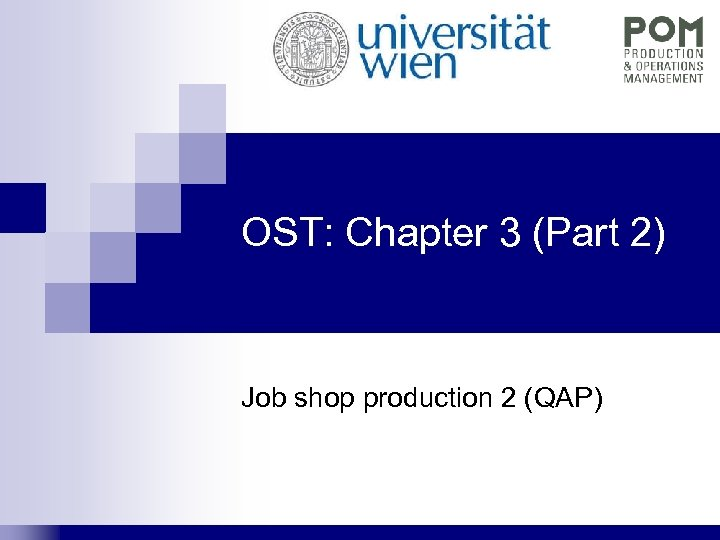OST: Chapter 3 (Part 2) Job shop production 2 (QAP)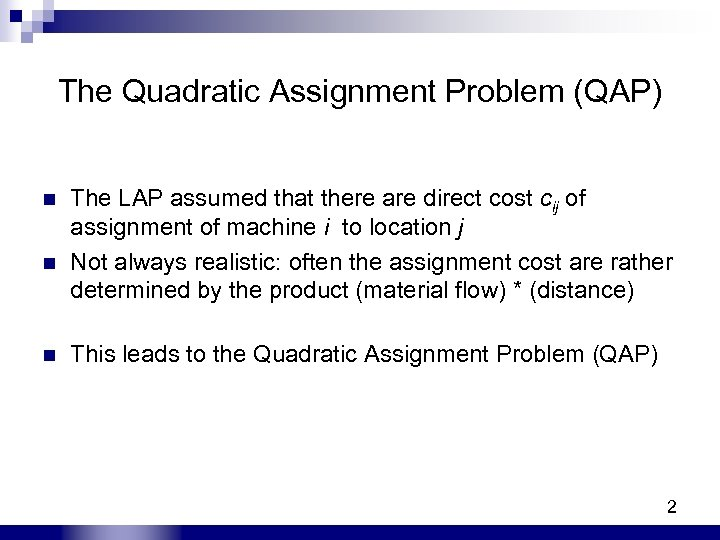The Quadratic Assignment Problem (QAP) n n n The LAP assumed that there are direct cost cij of assignment of machine i to location j Not always realistic: often the assignment cost are rather determined by the product (material flow) * (distance) This leads to the Quadratic Assignment Problem (QAP) 2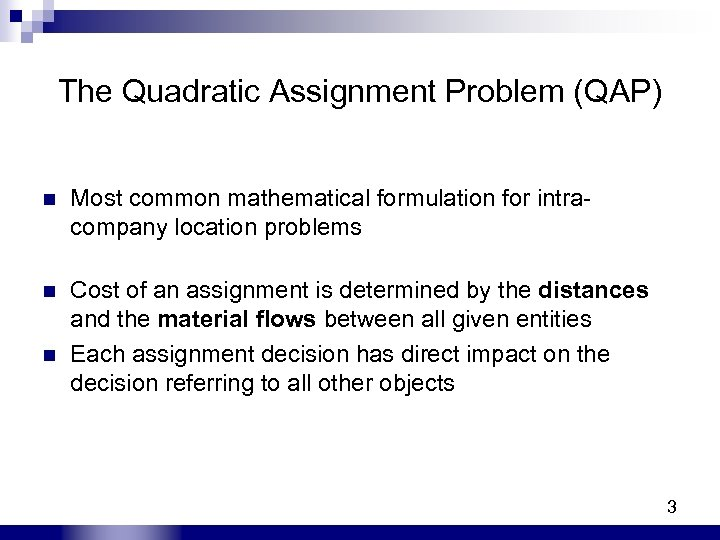The Quadratic Assignment Problem (QAP) n Most common mathematical formulation for intracompany location problems n Cost of an assignment is determined by the distances and the material flows between all given entities Each assignment decision has direct impact on the decision referring to all other objects n 3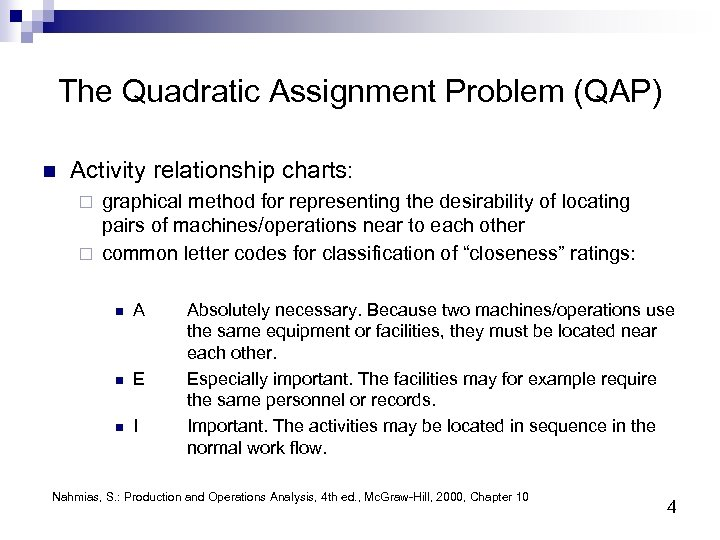The Quadratic Assignment Problem (QAP) n Activity relationship charts: graphical method for representing the desirability of locating pairs of machines/operations near to each other ¨ common letter codes for classification of “closeness” ratings: ¨ n A n E n I Absolutely necessary. Because two machines/operations use the same equipment or facilities, they must be located near each other. Especially important. The facilities may for example require the same personnel or records. Important. The activities may be located in sequence in the normal work flow. Nahmias, S. : Production and Operations Analysis, 4 th ed. , Mc. Graw-Hill, 2000, Chapter 10 4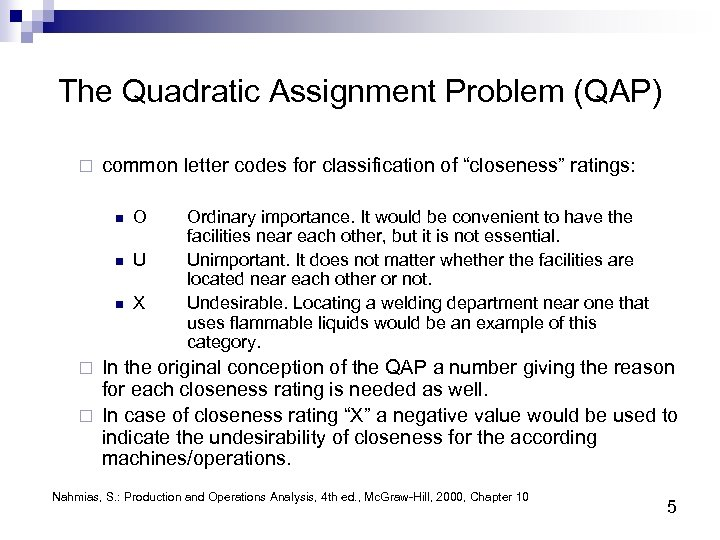The Quadratic Assignment Problem (QAP) ¨ common letter codes for classification of “closeness” ratings: n O n U n X Ordinary importance. It would be convenient to have the facilities near each other, but it is not essential. Unimportant. It does not matter whether the facilities are located near each other or not. Undesirable. Locating a welding department near one that uses flammable liquids would be an example of this category. In the original conception of the QAP a number giving the reason for each closeness rating is needed as well. ¨ In case of closeness rating “X” a negative value would be used to indicate the undesirability of closeness for the according machines/operations. ¨ Nahmias, S. : Production and Operations Analysis, 4 th ed. , Mc. Graw-Hill, 2000, Chapter 10 5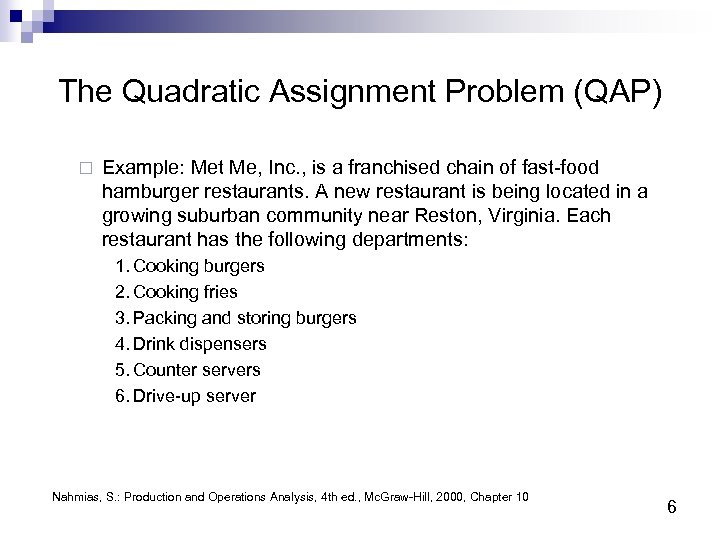The Quadratic Assignment Problem (QAP) ¨ Example: Met Me, Inc. , is a franchised chain of fast-food hamburger restaurants. A new restaurant is being located in a growing suburban community near Reston, Virginia. Each restaurant has the following departments: 1. Cooking burgers 2. Cooking fries 3. Packing and storing burgers 4. Drink dispensers 5. Counter servers 6. Drive-up server Nahmias, S. : Production and Operations Analysis, 4 th ed. , Mc. Graw-Hill, 2000, Chapter 10 6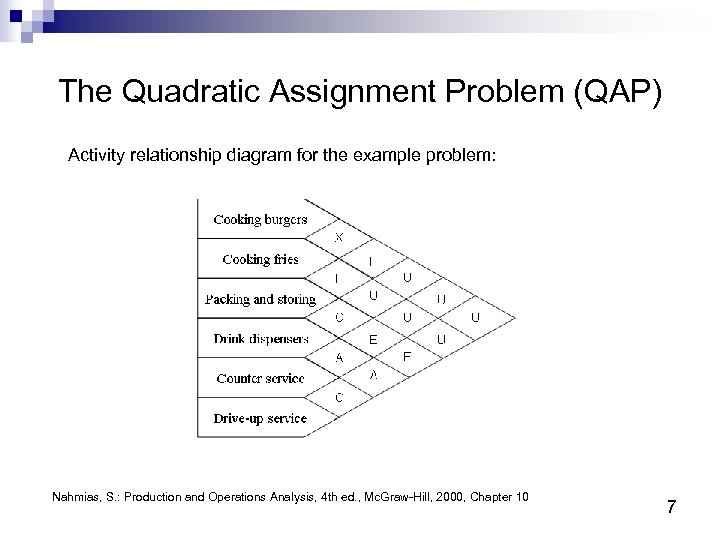The Quadratic Assignment Problem (QAP) Activity relationship diagram for the example problem: Nahmias, S. : Production and Operations Analysis, 4 th ed. , Mc. Graw-Hill, 2000, Chapter 10 7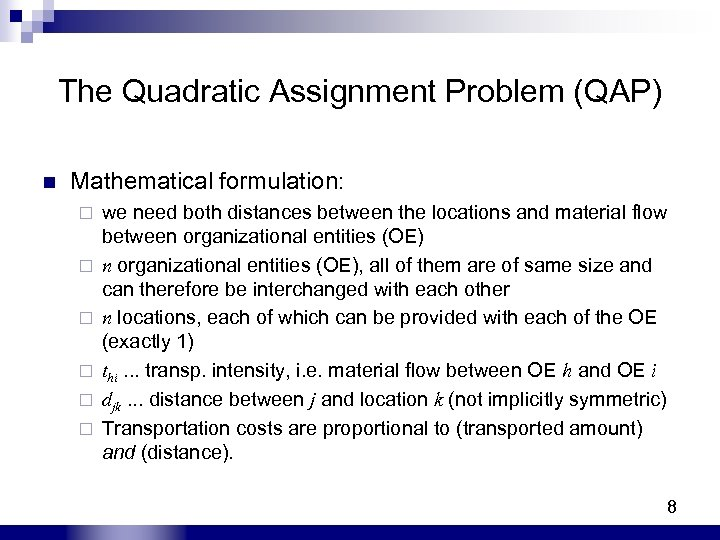The Quadratic Assignment Problem (QAP) n Mathematical formulation: ¨ ¨ ¨ we need both distances between the locations and material flow between organizational entities (OE), all of them are of same size and can therefore be interchanged with each other n locations, each of which can be provided with each of the OE (exactly 1) thi. . . transp. intensity, i. e. material flow between OE h and OE i djk. . . distance between j and location k (not implicitly symmetric) Transportation costs are proportional to (transported amount) and (distance). 8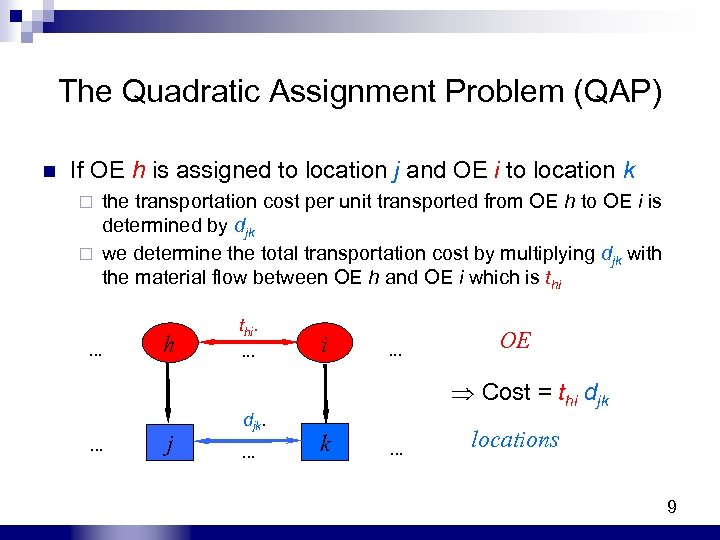The Quadratic Assignment Problem (QAP) n If OE h is assigned to location j and OE i to location k the transportation cost per unit transported from OE h to OE i is determined by djk ¨ we determine the total transportation cost by multiplying djk with the material flow between OE h and OE i which is thi ¨ . . . h thi. . i . . . OE Cost = thi djk . . . j djk. . k . . . locations 9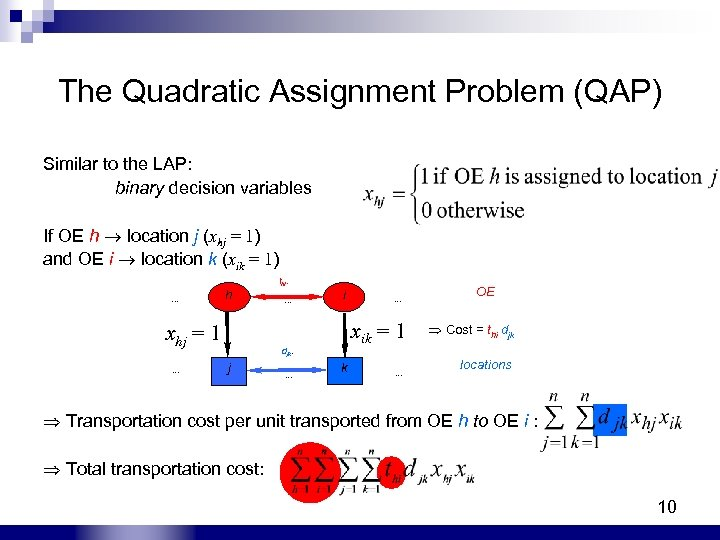The Quadratic Assignment Problem (QAP) Similar to the LAP: binary decision variables If OE h location j (xhj = 1) and OE i location k (xik = 1). . . h xhj = 1. . . thi. . i xik = 1 djk. j . . . k . . . OE Cost = thi djk locations Transportation cost per unit transported from OE h to OE i : Total transportation cost: 10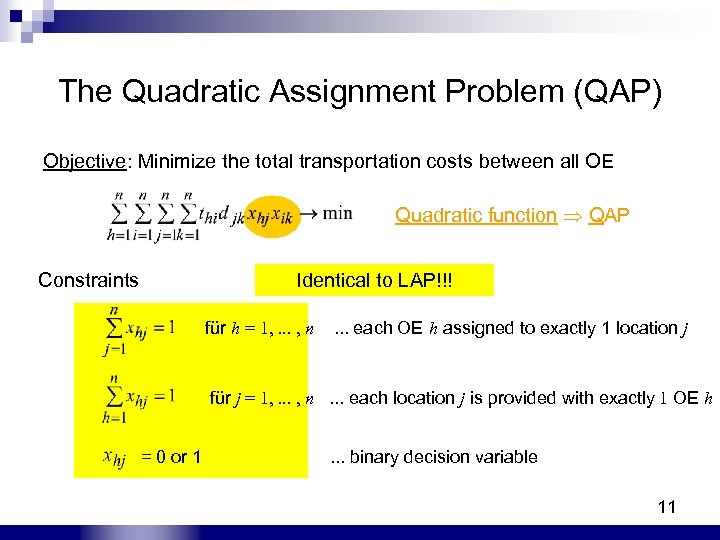The Quadratic Assignment Problem (QAP) Objective: Minimize the total transportation costs between all OE Quadratic function QAP Constraints Identical to LAP!!! für h = 1, . . . , n . . . each OE h assigned to exactly 1 location j für j = 1, . . . , n . . . each location j is provided with exactly 1 OE h = 0 or 1 . . . binary decision variable 11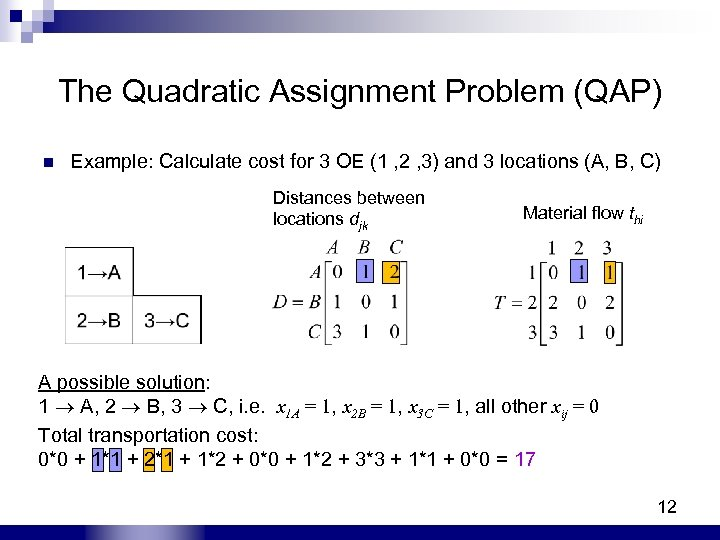The Quadratic Assignment Problem (QAP) n Example: Calculate cost for 3 OE (1 , 2 , 3) and 3 locations (A, B, C) Distances between locations djk Material flow thi A B C A possible solution: 1 A, 2 B, 3 C, i. e. x 1 A = 1, x 2 B = 1, x 3 C = 1, all other xij = 0 Total transportation cost: 0*0 + 1*1 + 2*1 + 1*2 + 0*0 + 1*2 + 3*3 + 1*1 + 0*0 = 17 12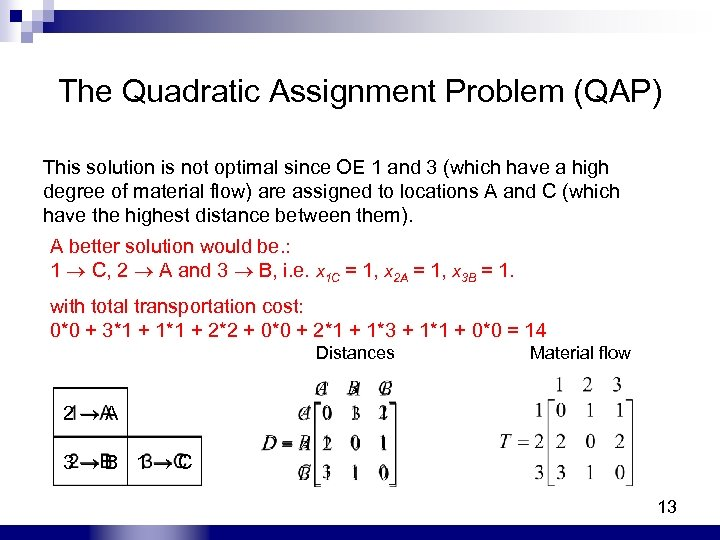The Quadratic Assignment Problem (QAP) This solution is not optimal since OE 1 and 3 (which have a high degree of material flow) are assigned to locations A and C (which have the highest distance between them). A better solution would be. : 1 C, 2 A and 3 B, i. e. x 1 C = 1, x 2 A = 1, x 3 B = 1. with total transportation cost: 0*0 + 3*1 + 1*1 + 2*2 + 0*0 + 2*1 + 1*3 + 1*1 + 0*0 = 14 Distances Material flow 2 A 3 B 1 C 13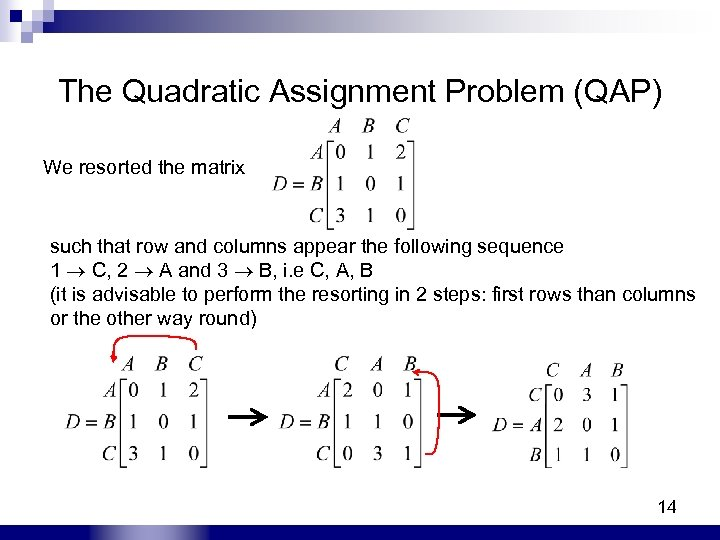The Quadratic Assignment Problem (QAP) We resorted the matrix such that row and columns appear the following sequence 1 C, 2 A and 3 B, i. e C, A, B (it is advisable to perform the resorting in 2 steps: first rows than columns or the other way round) 14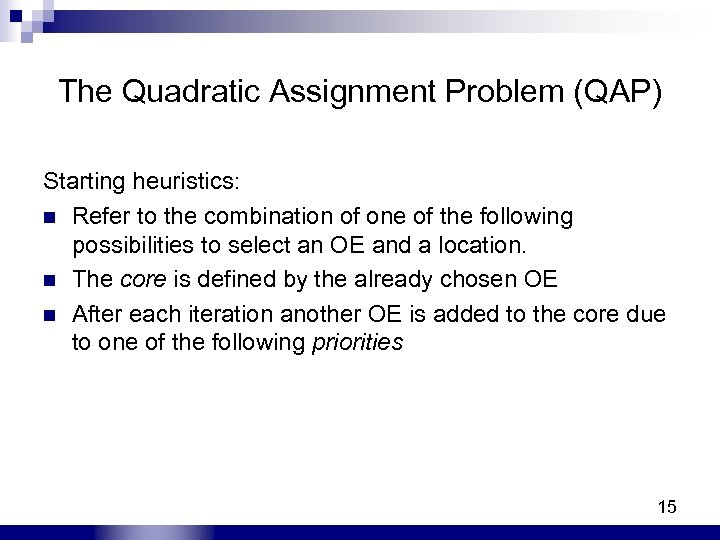The Quadratic Assignment Problem (QAP) Starting heuristics: n Refer to the combination of one of the following possibilities to select an OE and a location. n The core is defined by the already chosen OE n After each iteration another OE is added to the core due to one of the following priorities 15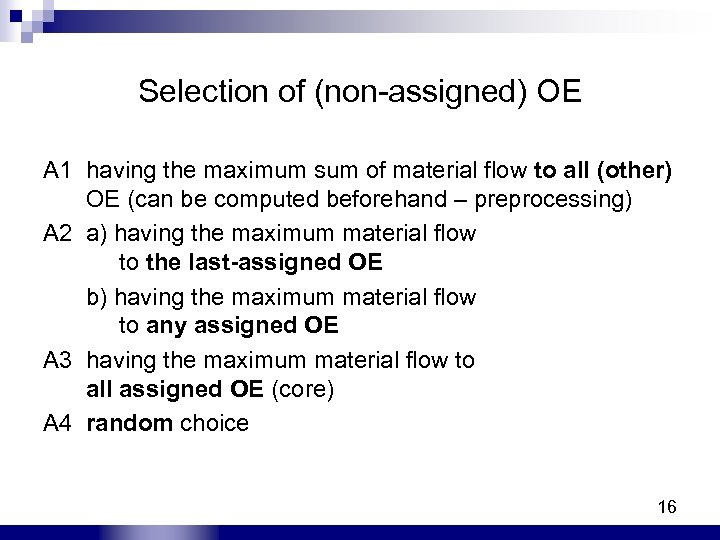Selection of (non-assigned) OE A 1 having the maximum sum of material flow to all (other) OE (can be computed beforehand – preprocessing) A 2 a) having the maximum material flow to the last-assigned OE b) having the maximum material flow to any assigned OE A 3 having the maximum material flow to all assigned OE (core) A 4 random choice 16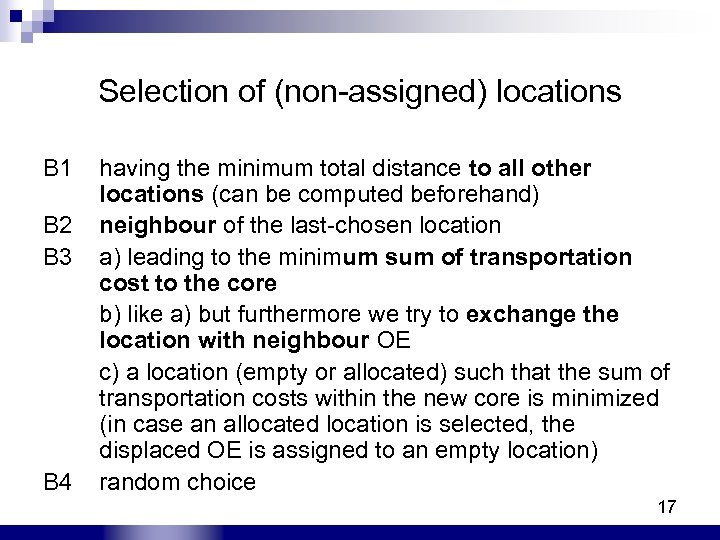Selection of (non-assigned) locations B 1 B 2 B 3 B 4 having the minimum total distance to all other locations (can be computed beforehand) neighbour of the last-chosen location a) leading to the minimum sum of transportation cost to the core b) like a) but furthermore we try to exchange the location with neighbour OE c) a location (empty or allocated) such that the sum of transportation costs within the new core is minimized (in case an allocated location is selected, the displaced OE is assigned to an empty location) random choice 17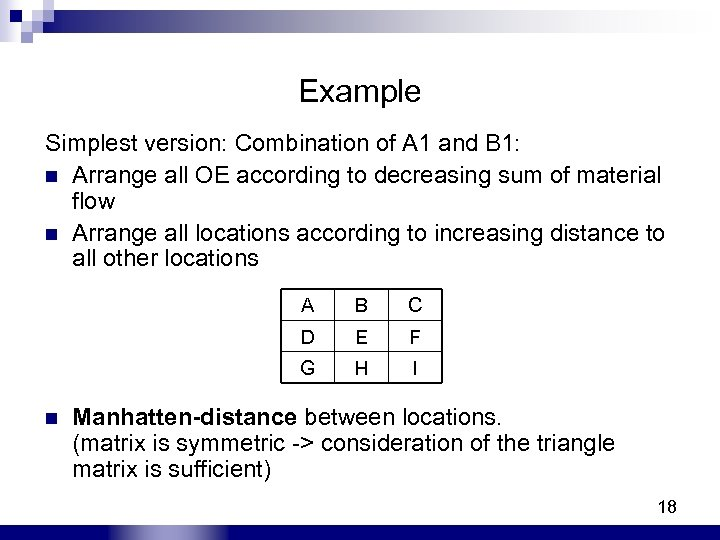Example Simplest version: Combination of A 1 and B 1: n Arrange all OE according to decreasing sum of material flow n Arrange all locations according to increasing distance to all other locations A B C D E F G H I n Manhatten-distance between locations. (matrix is symmetric -> consideration of the triangle matrix is sufficient) 18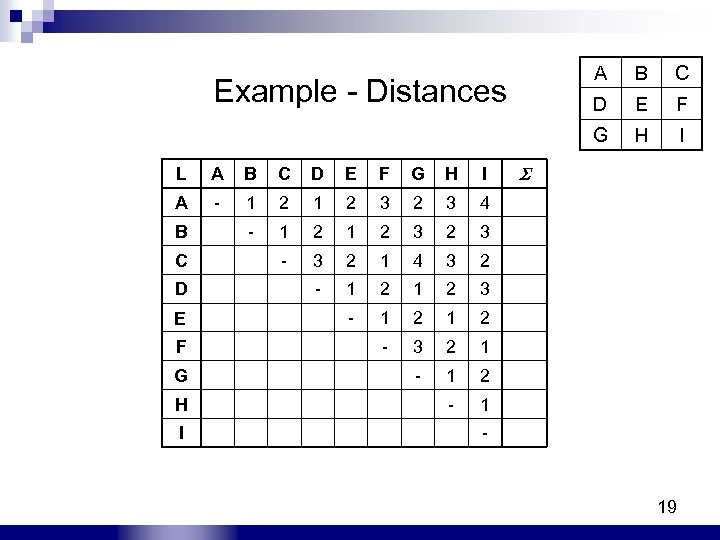A B C Example - Distances D E F G H I L A B C D E F G H I A - 1 2 3 2 3 4 B - 1 2 3 2 3 C - 3 2 1 4 3 2 D - 1 2 3 E - 1 2 F - 3 2 1 G - 1 2 H - 1 I - 19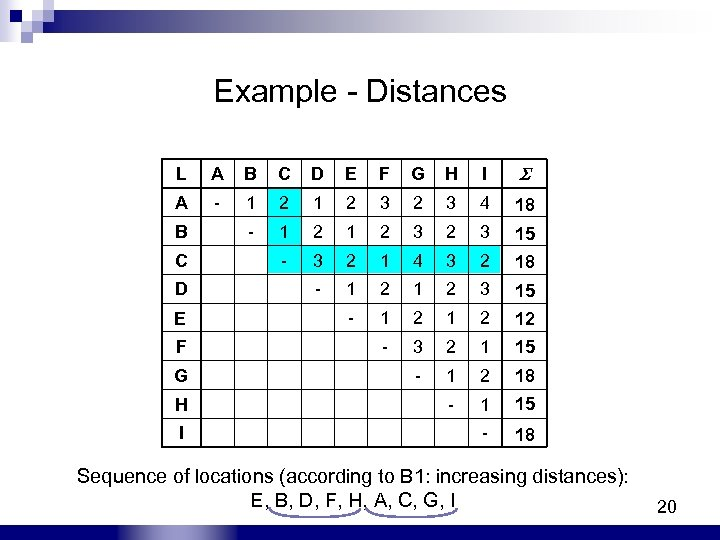Example - Distances L A B C D E F G H I A - 1 2 3 2 3 4 18 B - 1 2 3 2 3 15 C - 3 2 1 4 3 2 18 D - 1 2 3 15 E - 1 2 12 F - 3 2 1 15 G - 1 2 18 H - 1 15 I - 18 Sequence of locations (according to B 1: increasing distances): E, B, D, F, H, A, C, G, I 20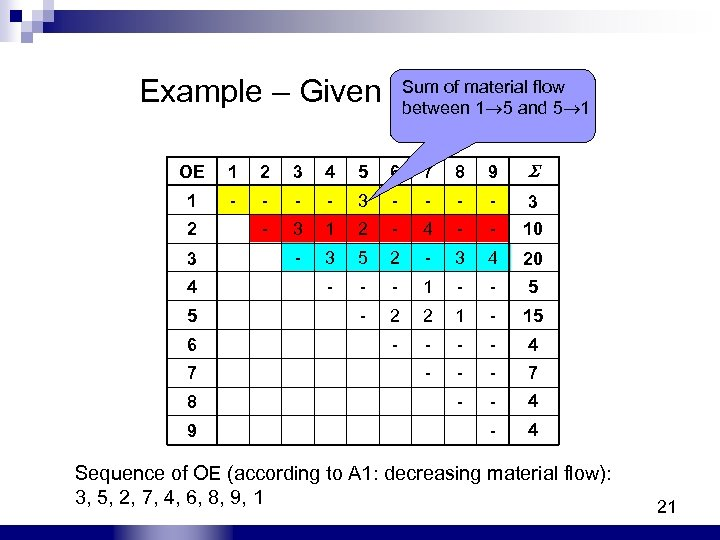Sum of material flow Example – Given Material Flow between 1 5 and 5 1 OE 1 2 3 4 5 6 7 8 9 1 - - - - 3 2 - 3 1 2 - 4 - - 10 3 - 3 5 2 - 3 4 20 4 - - - 1 - - 5 5 - 2 2 1 - 15 6 - - 4 7 - - - 7 8 - - 4 9 - 4 Sequence of OE (according to A 1: decreasing material flow): 3, 5, 2, 7, 4, 6, 8, 9, 1 21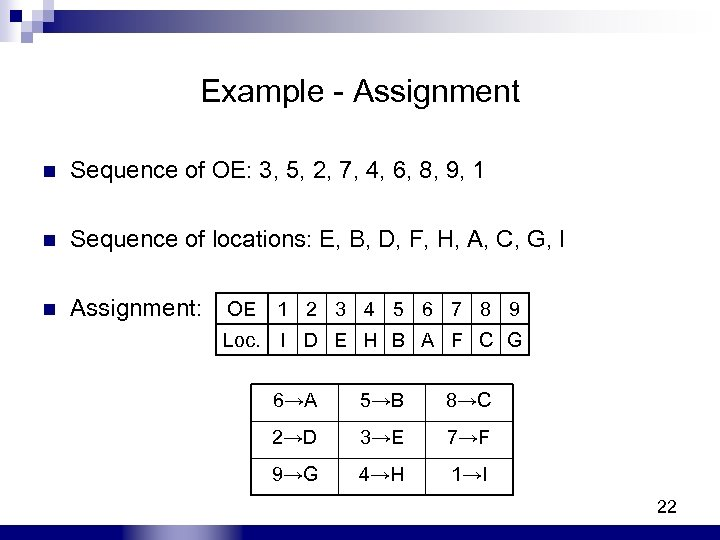Example - Assignment n Sequence of OE: 3, 5, 2, 7, 4, 6, 8, 9, 1 n Sequence of locations: E, B, D, F, H, A, C, G, I n Assignment: OE 1 2 3 4 5 6 7 8 9 Loc. I D E H B A F C G 6→A 5→B 8→C 2→D 3→E 7→F 9→G 4→H 1→I 22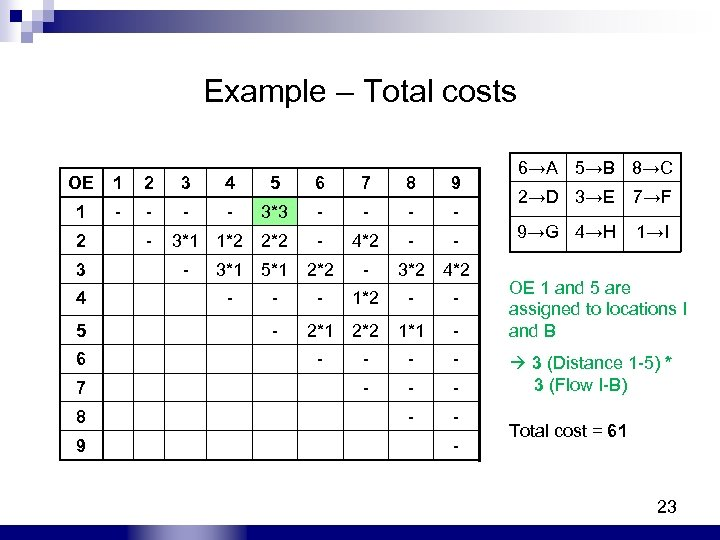Example – Total costs OE 1 2 3 4 5 6 7 8 9 1 - - 3*3 - - 2 - 2*2 - 4*2 - - 3*1 5*1 2*2 - 3*2 4 - - - 1*2 - - 5 - 2*1 2*2 1*1 - 6 - - 7 - - - 8 - - 9 - 3*1 1*2 6→A 5→B 8→C 2→D 3→E 7→F 9→G 4→H →I 1 OE 1 and 5 are assigned to locations I and B 3 (Distance 1 -5) * 3 (Flow I-B) Total cost = 61 23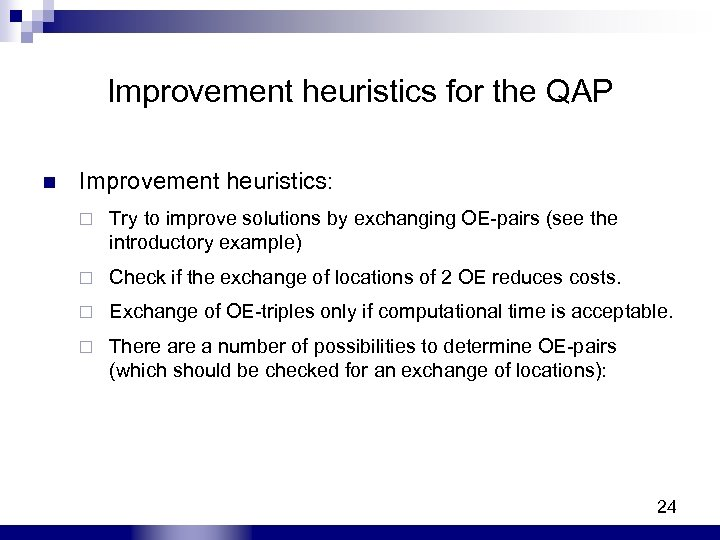Improvement heuristics for the QAP n Improvement heuristics: ¨ Try to improve solutions by exchanging OE-pairs (see the introductory example) ¨ Check if the exchange of locations of 2 OE reduces costs. ¨ Exchange of OE-triples only if computational time is acceptable. ¨ There a number of possibilities to determine OE-pairs (which should be checked for an exchange of locations): 24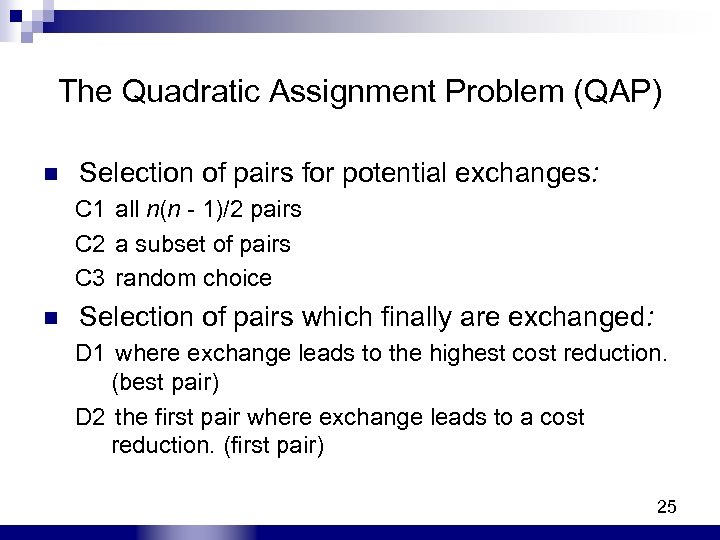The Quadratic Assignment Problem (QAP) n Selection of pairs for potential exchanges: C 1 all n(n - 1)/2 pairs C 2 a subset of pairs C 3 random choice n Selection of pairs which finally are exchanged: D 1 where exchange leads to the highest cost reduction. (best pair) D 2 the first pair where exchange leads to a cost reduction. (first pair) 25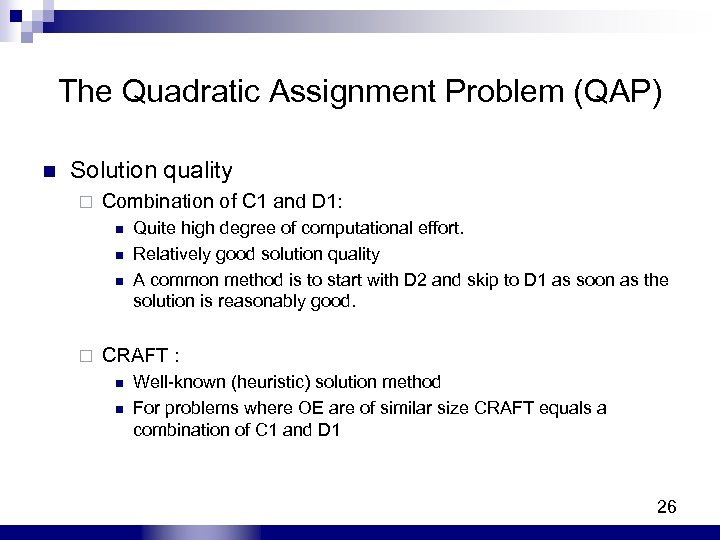The Quadratic Assignment Problem (QAP) n Solution quality ¨ Combination of C 1 and D 1: n n n ¨ Quite high degree of computational effort. Relatively good solution quality A common method is to start with D 2 and skip to D 1 as soon as the solution is reasonably good. CRAFT : n n Well-known (heuristic) solution method For problems where OE are of similar size CRAFT equals a combination of C 1 and D 1 26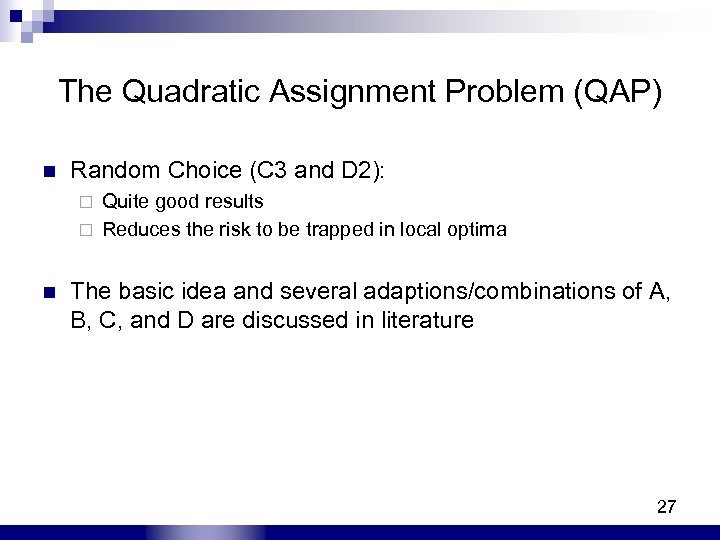The Quadratic Assignment Problem (QAP) n Random Choice (C 3 and D 2): Quite good results ¨ Reduces the risk to be trapped in local optima ¨ n The basic idea and several adaptions/combinations of A, B, C, and D are discussed in literature 27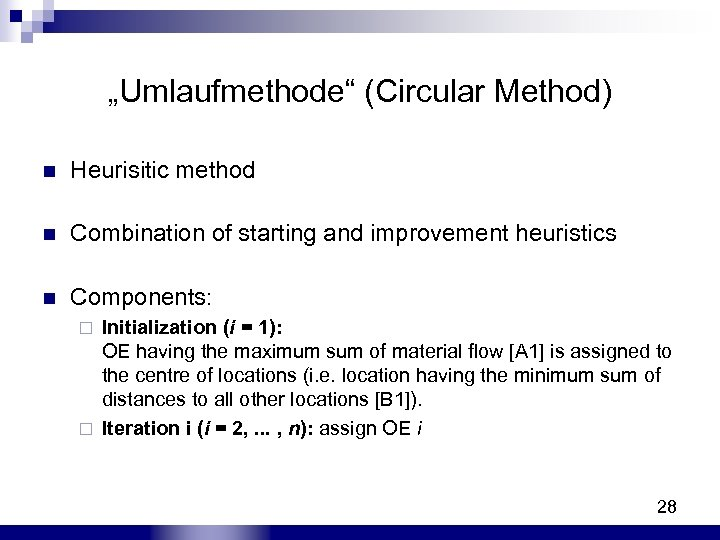„Umlaufmethode“ (Circular Method) n Heurisitic method n Combination of starting and improvement heuristics Components: n Initialization (i = 1): OE having the maximum sum of material flow [A 1] is assigned to the centre of locations (i. e. location having the minimum sum of distances to all other locations [B 1]). ¨ Iteration i (i = 2, . . . , n): assign OE i ¨ 28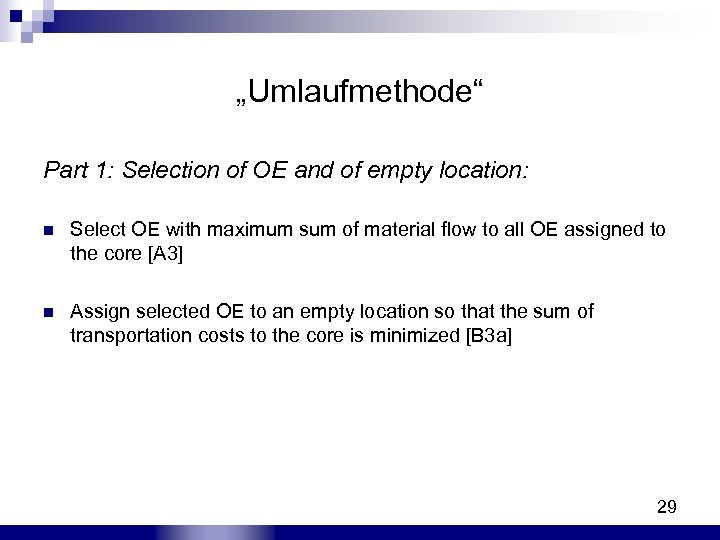„Umlaufmethode“ Part 1: Selection of OE and of empty location: n Select OE with maximum sum of material flow to all OE assigned to the core [A 3] n Assign selected OE to an empty location so that the sum of transportation costs to the core is minimized [B 3 a] 29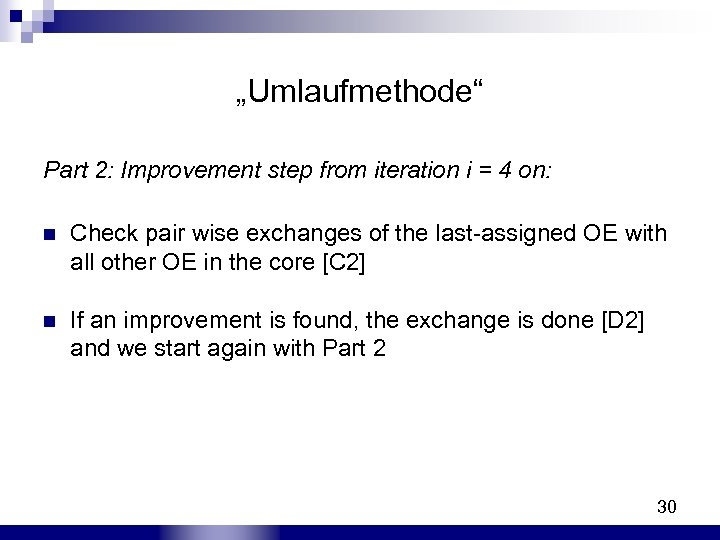„Umlaufmethode“ Part 2: Improvement step from iteration i = 4 on: n Check pair wise exchanges of the last-assigned OE with all other OE in the core [C 2] n If an improvement is found, the exchange is done [D 2] and we start again with Part 2 30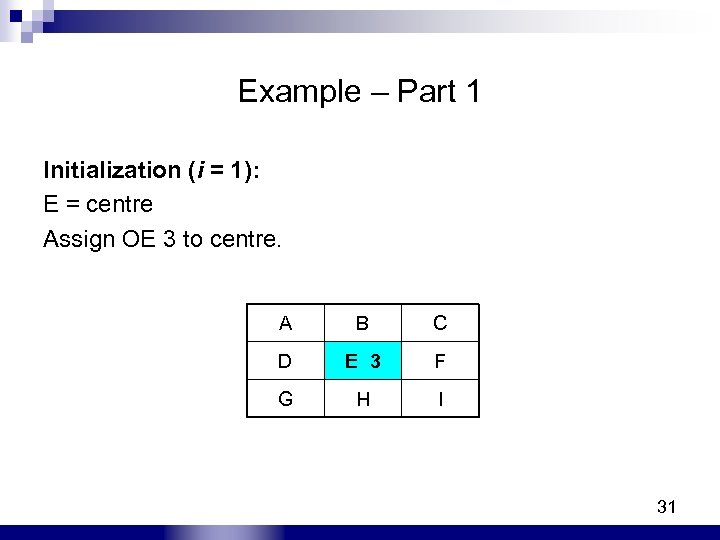Example – Part 1 Initialization (i = 1): E = centre Assign OE 3 to centre. A D G B E 3 H C F I 31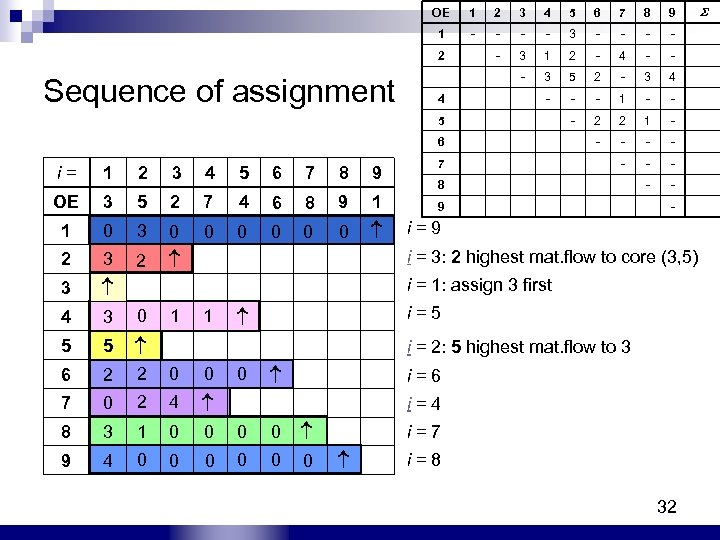OE 2 3 4 5 6 7 8 9 1 - - 3 - - 2 - 3 1 2 - 4 - - - 3 5 2 - 3 4 4 - - - 1 - - 5 - 2 2 1 - 6 Sequence of assignment 1 - - 7 - - - 8 - - 9 - i = 1 2 3 4 5 6 7 8 9 OE 3 5 2 7 4 6 8 9 1 1 0 3 0 0 0 i = 9 3 i = 3: 2 highest mat. flow to core (3, 5) 3 2 0 0 2 0 i = 1: assign 3 first 4 3 0 1 1 i = 5 5 5 i = 2: 5 highest mat. flow to 3 6 2 2 0 0 0 i = 6 7 0 2 4 i = 4 8 3 1 0 0 i = 7 9 4 0 0 0 i = 8 32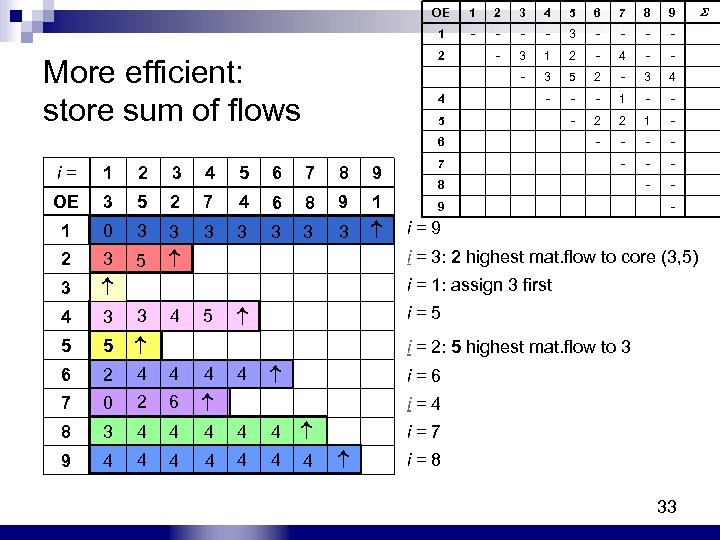OE 2 3 4 5 6 7 8 9 1 - - 3 - - 2 - 3 1 2 - 4 - - - 3 5 2 - 3 4 4 - - - 1 - - 5 - 2 2 1 - 6 More efficient: store sum of flows 1 - - 7 - - - 8 - - 9 - i = 1 2 3 4 5 6 7 8 9 OE 3 5 2 7 4 6 8 9 1 1 0 3 3 3 3 i = 9 3 i = 3: 2 highest mat. flow to core (3, 5) 3 5 3 3 2 3 i = 1: assign 3 first 4 3 3 4 5 i = 5 5 5 i = 2: 5 highest mat. flow to 3 6 2 4 4 i = 6 7 0 2 6 i = 4 8 3 4 4 4 i = 7 9 4 4 4 4 i = 8 33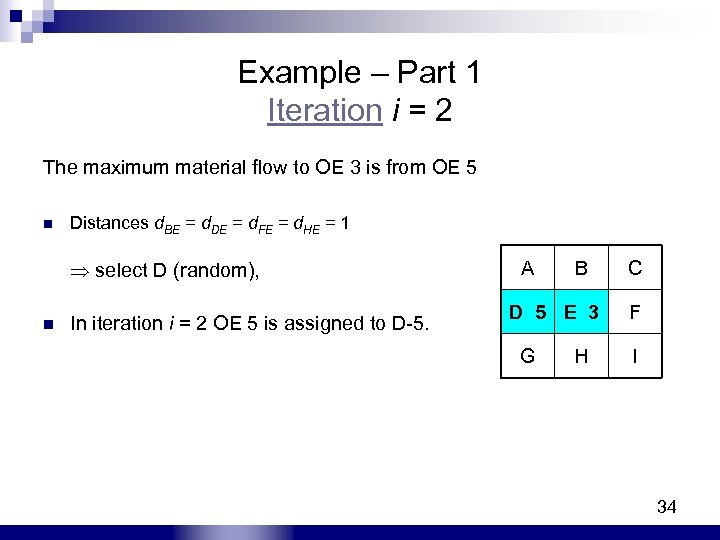Example – Part 1 Iteration i = 2 The maximum material flow to OE 3 is from OE 5 n Distances d. BE = d. DE = d. FE = d. HE = 1 select D (random), n In iteration i = 2 OE 5 is assigned to D-5. A B C D 5 E 3 F G H I 34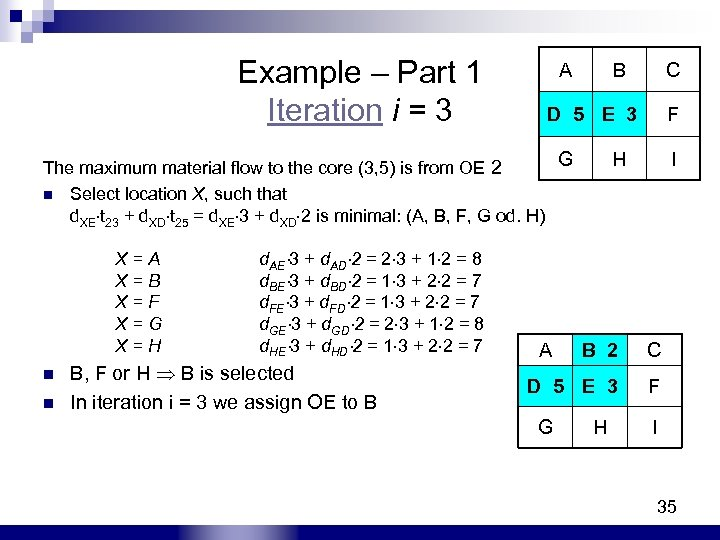Example – Part 1 Iteration i = 3 A B C D 5 E 3 F G H I The maximum material flow to the core (3, 5) is from OE 2 n Select location X, such that d. XE t 23 + d. XD t 25 = d. XE 3 + d. XD 2 is minimal: (A, B, F, G od. H) X = A d. AE 3 + d. AD 2 = 2 3 + 1 2 = 8 X = B d. BE 3 + d. BD 2 = 1 3 + 2 2 = 7 X = F d. FE 3 + d. FD 2 = 1 3 + 2 2 = 7 X = G d. GE 3 + d. GD 2 = 2 3 + 1 2 = 8 X = H d. HE 3 + d. HD 2 = 1 3 + 2 2 = 7 A B 2 C n n B, F or H B is selected In iteration i = 3 we assign OE to B D 5 E 3 F G H I 35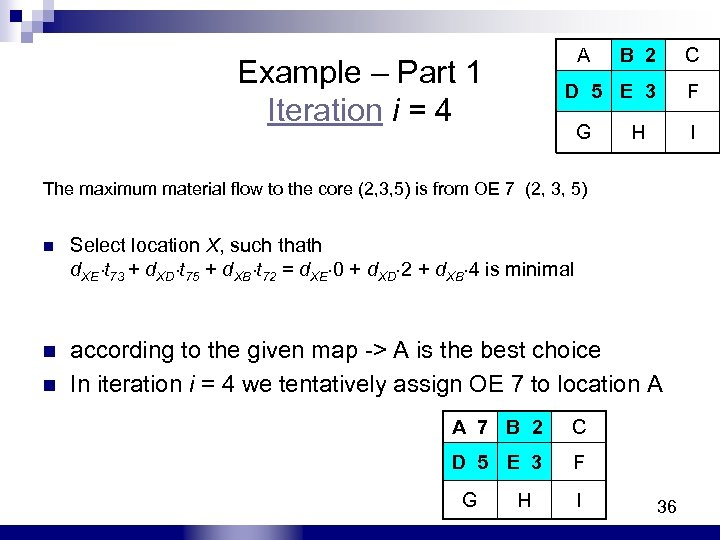Example – Part 1 Iteration i = 4 A B 2 C D 5 E 3 F G H I The maximum material flow to the core (2, 3, 5) is from OE 7 (2, 3, 5) n Select location X, such thath d. XE t 73 + d. XD t 75 + d. XB t 72 = d. XE 0 + d. XD 2 + d. XB 4 is minimal n according to the given map -> A is the best choice In iteration i = 4 we tentatively assign OE 7 to location A 7 B 2 C D 5 E 3 F G H I 36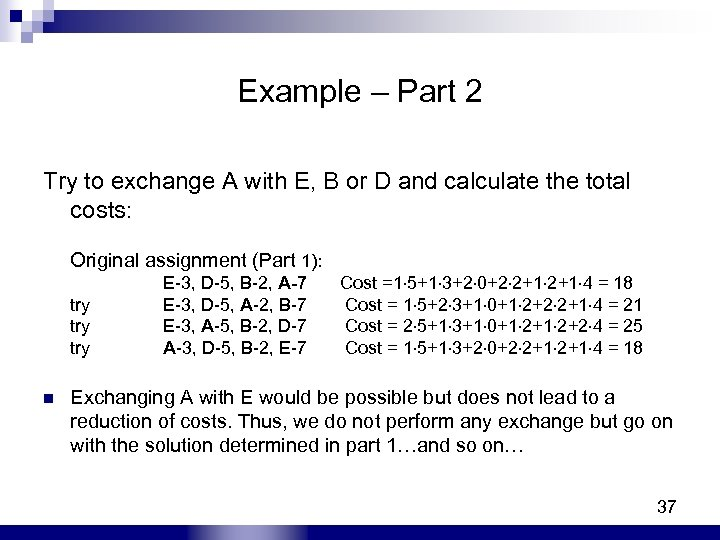Example – Part 2 Try to exchange A with E, B or D and calculate the total costs: Original assignment (Part 1): try try n E-3, D-5, B-2, A-7 E-3, D-5, A-2, B-7 E-3, A-5, B-2, D-7 A-3, D-5, B-2, E-7 Cost =1 5+1 3+2 0+2 2+1 4 = 18 Cost = 1 5+2 3+1 0+1 2+2 2+1 4 = 21 Cost = 2 5+1 3+1 0+1 2+2 4 = 25 Cost = 1 5+1 3+2 0+2 2+1 4 = 18 Exchanging A with E would be possible but does not lead to a reduction of costs. Thus, we do not perform any exchange but go on with the solution determined in part 1…and so on… 37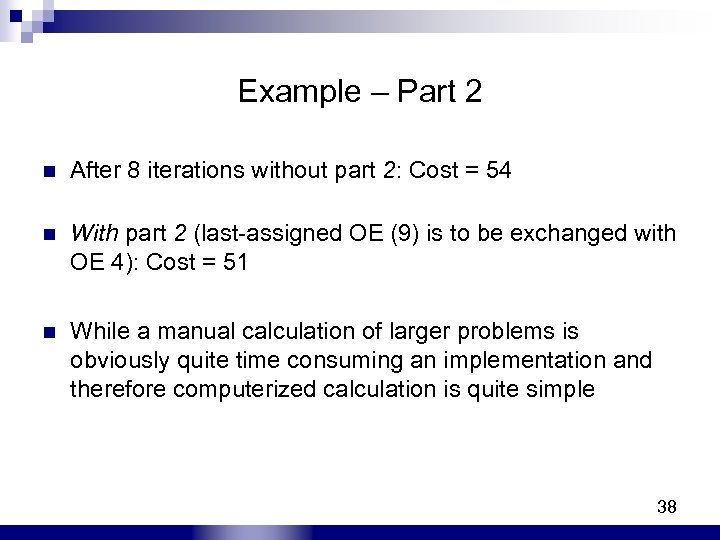Example – Part 2 n After 8 iterations without part 2: Cost = 54 n With part 2 (last-assigned OE (9) is to be exchanged with OE 4): Cost = 51 n While a manual calculation of larger problems is obviously quite time consuming an implementation and therefore computerized calculation is quite simple 38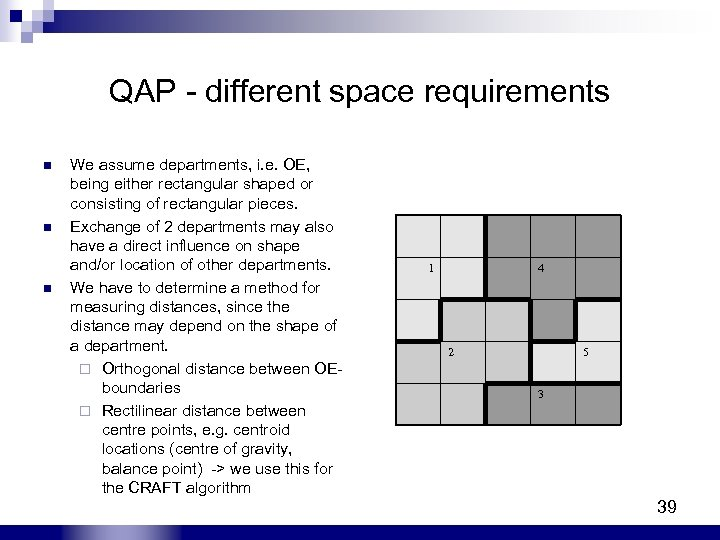QAP - different space requirements n n n We assume departments, i. e. OE, being either rectangular shaped or consisting of rectangular pieces. Exchange of 2 departments may also have a direct influence on shape and/or location of other departments. We have to determine a method for measuring distances, since the distance may depend on the shape of a department. ¨ Orthogonal distance between OEboundaries ¨ Rectilinear distance between centre points, e. g. centroid locations (centre of gravity, balance point) -> we use this for the CRAFT algorithm 1 4 2 5 3 39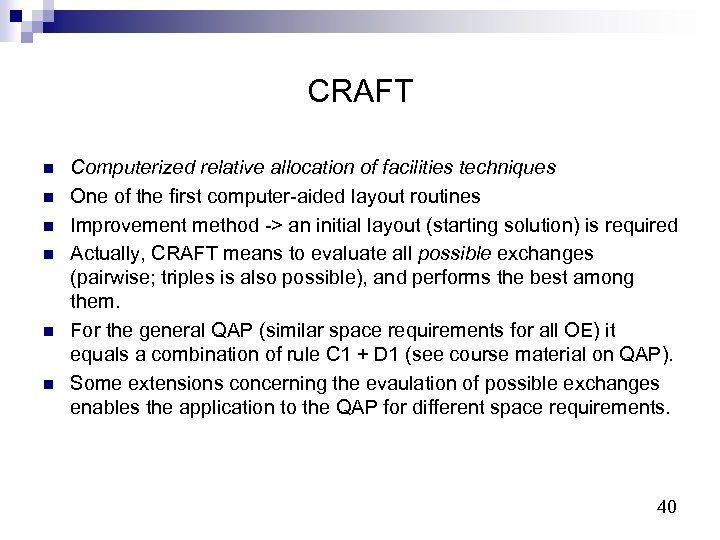CRAFT n n n Computerized relative allocation of facilities techniques One of the first computer-aided layout routines Improvement method -> an initial layout (starting solution) is required Actually, CRAFT means to evaluate all possible exchanges (pairwise; triples is also possible), and performs the best among them. For the general QAP (similar space requirements for all OE) it equals a combination of rule C 1 + D 1 (see course material on QAP). Some extensions concerning the evaulation of possible exchanges enables the application to the QAP for different space requirements. 40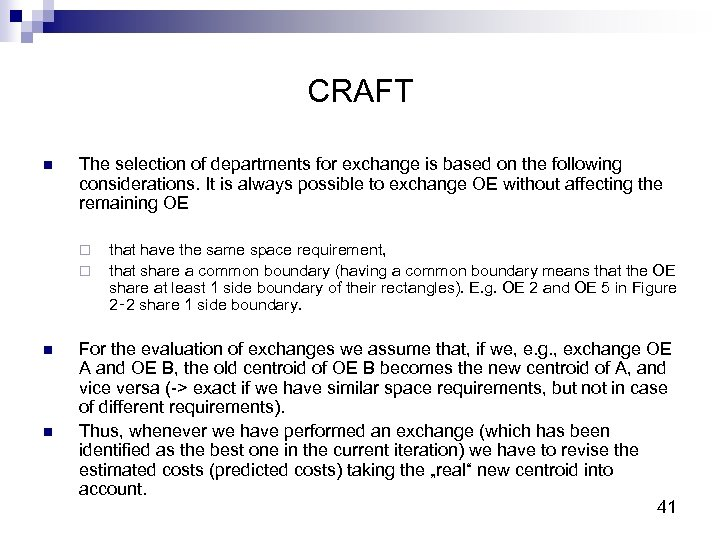CRAFT n The selection of departments for exchange is based on the following considerations. It is always possible to exchange OE without affecting the remaining OE ¨ ¨ n n that have the same space requirement, that share a common boundary (having a common boundary means that the OE share at least 1 side boundary of their rectangles). E. g. OE 2 and OE 5 in Figure 2‑ 2 share 1 side boundary. For the evaluation of exchanges we assume that, if we, e. g. , exchange OE A and OE B, the old centroid of OE B becomes the new centroid of A, and vice versa (-> exact if we have similar space requirements, but not in case of different requirements). Thus, whenever we have performed an exchange (which has been identified as the best one in the current iteration) we have to revise the estimated costs (predicted costs) taking the „real“ new centroid into account. 41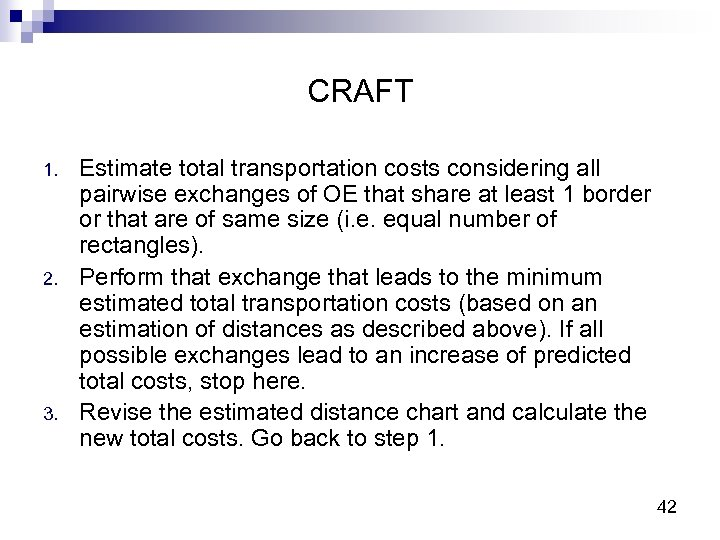CRAFT 1. 2. 3. Estimate total transportation costs considering all pairwise exchanges of OE that share at least 1 border or that are of same size (i. e. equal number of rectangles). Perform that exchange that leads to the minimum estimated total transportation costs (based on an estimation of distances as described above). If all possible exchanges lead to an increase of predicted total costs, stop here. Revise the estimated distance chart and calculate the new total costs. Go back to step 1. 42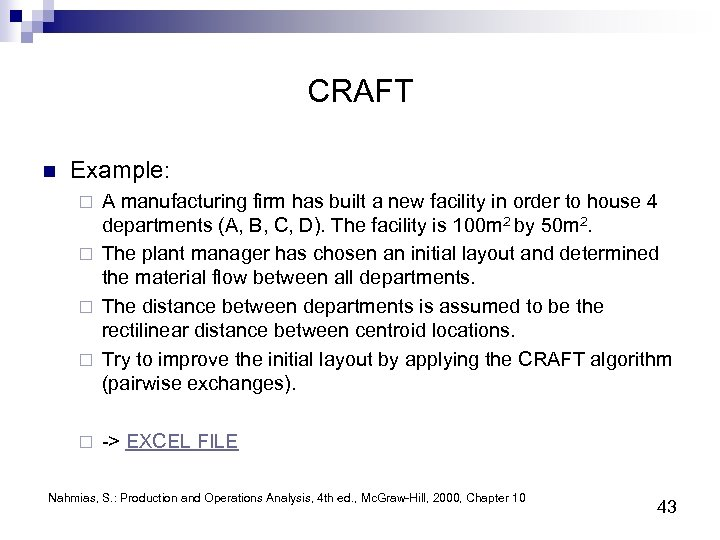CRAFT n Example: A manufacturing firm has built a new facility in order to house 4 departments (A, B, C, D). The facility is 100 m 2 by 50 m 2. ¨ The plant manager has chosen an initial layout and determined the material flow between all departments. ¨ The distance between departments is assumed to be the rectilinear distance between centroid locations. ¨ Try to improve the initial layout by applying the CRAFT algorithm (pairwise exchanges). ¨ ¨ -> EXCEL FILE Nahmias, S. : Production and Operations Analysis, 4 th ed. , Mc. Graw-Hill, 2000, Chapter 10 43